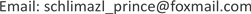1. 引言

2. 问题介绍

2017年，美国的波多黎各(Puerto Rico)遭遇最严重飓风袭击。飓风使该岛遭受严重破坏，造成2900多人死亡。狂风暴雨击倒了波多黎各80％的电线杆和所有输电线路，导致基本上所有岛屿都失去了电力。此外，风暴还破坏或摧毁了岛上大部分的蜂窝通信网络。岛上大部分地区的电力和电池服务中断持续数月，而在某些地区则更长。广泛的洪水阻塞并破坏了岛上的许多高速公路和道路，使得紧急服务地面车辆几乎不可能规划和导航他们的路线。波多黎各的数十个地区被孤立，没有通信。

3. 模型建立以及求解流程 [<xref ref-type="bibr" rid="hanspub.32155-ref2">2</xref>]3.1. 建立选址问题的数学模型

min ∑ j = 1 k ∑ x ∈ s j y i j ‖ X − m j ‖ (1)

s.t.

∑ i = 1 n y i j = 1 ( i = 1 , 2 , ⋯ , n ) (2)

m j = 1 ∑ i = 1 n y i j ∑ i = 1 n y i j X i ( j = 1 , 2 , ⋯ , K ) (3)

y i j = { 1 0 (4)

3.2. K-Means聚类算法求解流程

K-means算法的求解步骤如下  ：

STEP1：任意选择k个初始的聚类中心为 c 1 , c 2 , ⋯ , c k ；

STEP2：逐一将样本集 { X } 中的各个样本按照最小距离的原则分配给k个聚类中心的其中一个 c j ；

STEP3：由上计算新的聚类中心 c ′ j = 1 N j ∑ X ( j = 1 , 2 , ⋯ , k ) ，其中 N j 表示第j个聚类中心包含的样本个数；

STEP4：如果满足 c ′ j ≠ c j ，转至STEP2；否则即为算法收敛，结束。

4. 实验计算

d = 2 R arccos ( cos θ 1 cos θ 2 cos α + sin θ 1 sin θ 2 )

5. 结果分析

Comparison of clustering methods for geographic coordinates of container locatio

1−66.1418.24−66.9818.25
2−66.718.23−66.0718.31
3−67.0818.27−66.418.16